Next: CONCLUSION Up: CHIRPLET TRANSFORM SUBSPACES Previous: Nondilational Chirplet Transform

## Warbling Chirplet: Analysis of Signals of Oscillating Frequency

Suppose we choose a windowed sinusoidal FM signal for our mother chirplet. Such a signal has a frequency that periodically rises and falls (much like the vibrato of musical instruments or the wail of a police siren).

Within time-frequency space, conventional Doppler radar spectrograms treat the motion of objects as though their velocities (Doppler frequencies) were piecewise constant (constant over each of the short time intervals), whereas the chirplet transform attained a certain advantage by generalizing to a piecewise constant acceleration model.

Originally, we had further extended the linear FM chirplet bases to piecewise quadratic, and piecewise cubic FM - piecewise polynomial approximations to the time-frequency evolution of Doppler returns. However, looking more closely at the underlying physics of floating objects, which was our main motivation that led to our discovery of the CCT, we observed a somewhat sinusoidal evolution of the Doppler signals. If you have ever watched a cork bobbing up and down at the seaside, you would notice that it moves around in a circle with a distinct periodicity. It moves up and down, but it also moves horizontally. Looking out at a target with a radar, for example, we see the horizontal component of motion (which is essentially a scaled version of the Hilbert transform of the vertical movement). This sinusoidalhorizontal movement results in a sinusoidally varying frequency in the Doppler return.

We wish to end up with the instantaneous frequency of the basis function being given by:

f = (2f_mt+p)+f_c

where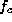is the center (carrier) frequency, p (which varies on the interval from 0 to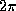) is the relative position of one of the peak epochs in frequency, with respect to the origin, and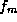is the modulation frequency. If we are analyzing a discrete signal, s[nT], we also note that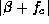must be less than 1/2, otherwise the frequency modulation is not bounded by the Nyquist limit.

Integrating to get the phase, we get:

= sin(2f_m t +p)f_m + 2f_ct

which gives us the family of chirplets defined by:

g_f_m,,f_c = A e^j(sin(2f_m t +p)f_m) + j2f_ct

which may be appropriately windowed, such as with a Gaussian, as was done in (3).

In Fig. 13, we show four examples taken from a family of chirplets that were derived from a warbling mother chirplet. We show them in both the time-domain, and the TF domain, annotated in terms of the pendulum model described now.

Figure: FIGURE GOES SOMEWHERE IN THIS GENERAL VICINITY

Pendulums swinging to and fro in front of a radar (assume the amplitude of the swing is small compared to the length of the string) produce a signal which is very similar to that produced by radar returns from floating objects. Suppose the velocity of a pendulum, as a function of time, is given by:

v = (2f_m t)

(the position is given by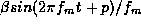)

The demodulated radar Doppler signal would then be given by

e^jsin(2f_m t +p)f_m

which may be analyzed using the family of chirplets given by (24).

A pendulum with a long string, swinging with large amplitude in front of the radar will produce a time series which, will have most of its energy in the upper left hand portion of the space (lowand high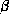). A density plot of the transform, computed from the time series will show a strong peak in the upper left region, with the peak located at the coordinates corresponding to the particular frequency of swinging () and amplitude. A pendulum with a small swing, and a short length, will appear as an energy concentration in the lower right corner of the pendulum parameter space.

Figure 14

Figure: FIGURE GOES SOMEWHERE IN THIS GENERAL VICINITY

shows where four pendulums would appear as peaks in this pendulum parameter space. Each of these four points in the space corresponds to the four examples of Fig. 13.

In Fig. 15

Figure: FIGURE GOES SOMEWHERE IN THIS GENERAL VICINITY

we show the STFT computed from an actual radar return from a pendulum.

Using the warbling mother chirplet, we also computed the ``dilation-dilation'' (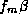) plane of the chirplet transform (Fig. 16) for the pendulum data.

Figure: FIGURE GOES SOMEWHERE IN THIS GENERAL VICINITY

The members of the chirplet family given by (24) may be regarded as being related to each other by affine coordinate transformations in the time-frequency plane if we use the rather abstract notion of instantaneous frequency. Consider four functions from (24), denoted A, B, C, and D, corresponding to the four signals depicted in Fig. 13 within the plane formed by time and instantaneous frequency. While there is no way to actually calculate this plane, we can consider these functions as being defined by sinusoids in this plane. Then A is a frequency-dilated version of C, and B is a frequency-dilated version of D. While there is no practical means of dilating frequency without contracting time, or vice-versa, we may denote the abstract operator:

C_0,0,0,0,0,_t _f \: g(t)

as an operator that would magnify the time-frequency distribution of g(t).

When we write, for example,

C_0,0,0,0,0,2 \; g(t),

we mean to replace g(t) with another function that occupies twice the area in the TF plane. In general, such a function probably does not exist. We noted, in the case of the prolate chirplet family, that we could, however, vary the time-bandwidth product of the tiling by replacing the family of mother chirplets with a new family that had a different value of NW. By similar reasoning, within the context of the warbling chirplet we interpret (28) to mean ``replace g(t) with a new sinusoidal-FM function that has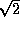times the modulation index and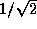times the modulation frequency'', so that, we obtain an equal dilation byalong each of the time and instantaneous frequency axes. The result is a dilation of both the time and instantaneous frequency axes by a factor of. The law of composition, identity, and inverse, within this six-parameter ``group'' is given by the usual two-dimensional affine group lawartin.

Therefore, we may write the warbling chirplet transform in terms of the six affine coordinate transformations in the TF plane:

S_f_m,,f_c =

C_0,f_c,1f_m ,0,0,f_m \: g(t) | s(t)

and refer to the subspace (Fig. 16) defined along theandaxes as the ``dilation-dilation'' plane, or the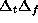plane.Next: CONCLUSION Up: CHIRPLET TRANSFORM SUBSPACES Previous: Nondilational Chirplet Transform

Steve Mann
Thu Jan 8 19:50:27 EST 1998